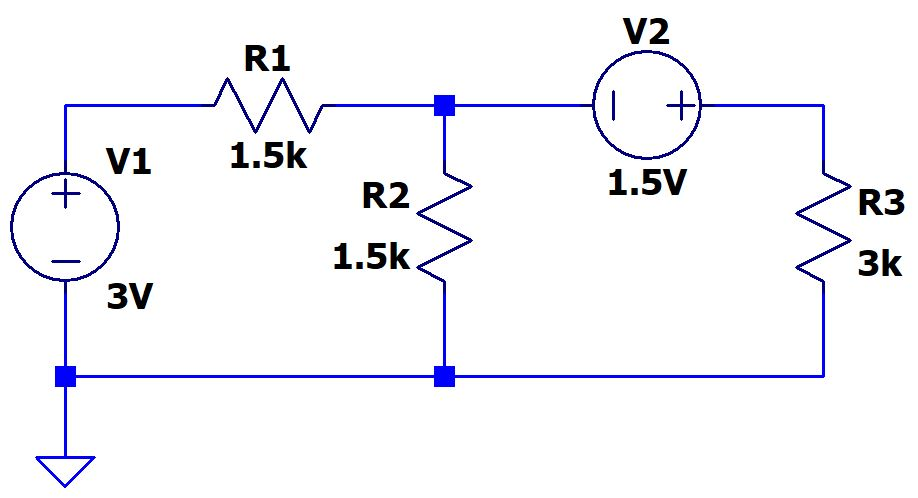ENGR338 Digital Electronics Laboratory
Lab 1 Review Superposition, Thevenin's Equivalent Circuit, and LTSpice

Objectives:
1. Review the superposition and the Thevenin equivalent theorem.
2. Review LTSpice.

Lecture videos on:
Lectures of Thevenin's equivalent circuit and Superposition.
Lecture of how to use LTSpice Code

Task 1: Review Superposition and Spice (50 points). Grading rubric: Spice code (10 points), hand calculation (10 points), simulation results (10 points), writing/formatting (20 points).
(Build the circuit in Spice code ONLY. Do not use symbols and schematics)
Use the superposition theory to calculate all the voltages and currents in the following circuit (Label it properly, show the calculation process for the credit). Verify all the values using LTSpice (Spice code only, do not use symbols/schematics).Task 2: Review Thevenin's Equivalent Circuit and RC time delay
(50 points). Grading rubric: Spice code (10 points), hand calculation (10 points), simulation results (10 points), writing/formatting (20 points).
(Build the circuit in Spice code ONLY. Do not use symbols and schematics)

Treat the 4nF CL as the load. Convert the circuit on the left hand side of CL into its Thevenin's equivalent circuit.
I didn't assign a PULSE function to the voltage source. You can define the parameters of the PULSE() function on your own as long as you can show a nice time delay of your input/output and the output is fully charged to the full voltage during the periods. The arguments in the PULSE() function are:
PULSE (LowVoltage   HighVoltage   Delay   RisingTime   FallingTime   OnTime   Period).

Show the calculation process of the time delay at Vout compare to Vin and the simulation results for the credit.--------------------------This the end of the lab